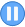﻿

## GMAT Practice Question Set #1 (Question 1-3)

GMAT Integrated Reasoning  >  Two-Part Analysis Question # 1: Number of Computer Science Teachers
 Time 0 0 : 0 0 : 0 0Between 2008 and 2012, the number of computer science teachers decreased despite a jump in computer science majors from 400 in 2008.
In the given expressions, T and S represent the percent change in the number of teachers and computer science students, respectively, over the four years and R represents the the number of computer science students per teacher in 2008.
Percent change in a quantity X is calculated using the formula
((X_(recent)- X_(old) ) / X_(old)) * 100.
Select the expression that represents the number of teachers in 2012 and select the expression that represents the number of computer science students per teacher in 2012.# of Teachers in 2012 # of Computer Science Students per Teacher in 2012(400/R)((100-T)/100)R((100 + S) / (100 + T))1/RR((100-T)/(100+S))400/R ((100+T) / 100)## Video Explanation Part 3 of 3: Summarizing It All Up

 GMATTM is a registered trademark of the Graduate Management Admission CouncilTM. The Graduate Management Admission CouncilTM does not endorse, nor is it affiliated in any way with the owner or any content of this web site.